# Chart Visualization¶

This section demonstrates visualization through charting. For information on visualization of tabular data please see the section on Table Visualization.

We use the standard convention for referencing the matplotlib API:

```In : import matplotlib.pyplot as plt

In : plt.close("all")
```

We provide the basics in pandas to easily create decent looking plots. See the ecosystem section for visualization libraries that go beyond the basics documented here.

Note

All calls to `np.random` are seeded with 123456.

## Basic plotting: `plot`¶

We will demonstrate the basics, see the cookbook for some advanced strategies.

The `plot` method on Series and DataFrame is just a simple wrapper around `plt.plot()`:

```In : ts = pd.Series(np.random.randn(1000), index=pd.date_range("1/1/2000", periods=1000))

In : ts = ts.cumsum()

In : ts.plot();
```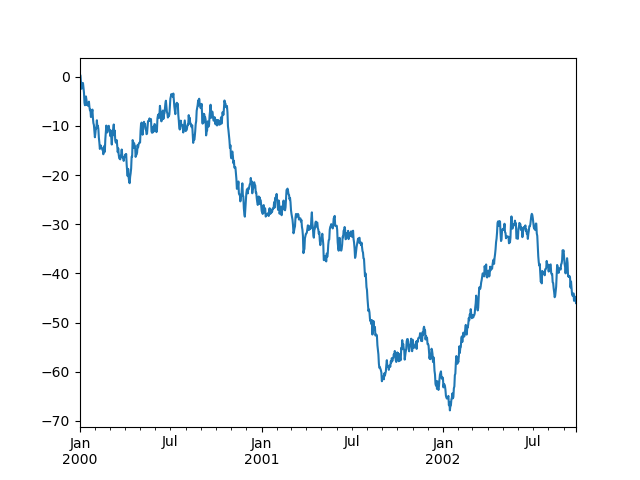If the index consists of dates, it calls `gcf().autofmt_xdate()` to try to format the x-axis nicely as per above.

On DataFrame, `plot()` is a convenience to plot all of the columns with labels:

```In : df = pd.DataFrame(np.random.randn(1000, 4), index=ts.index, columns=list("ABCD"))

In : df = df.cumsum()

In : plt.figure();

In : df.plot();
```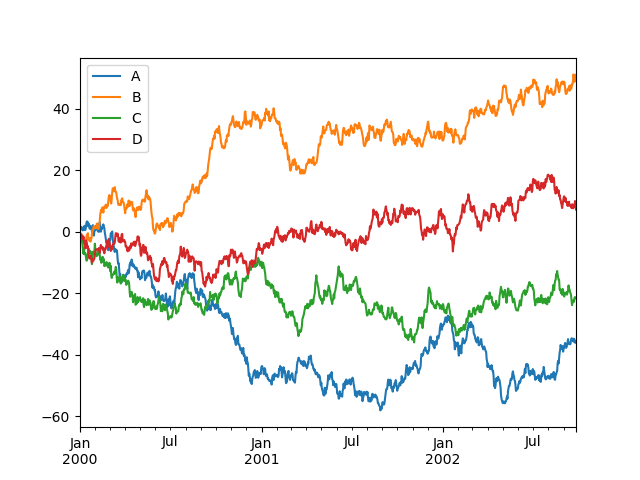You can plot one column versus another using the `x` and `y` keywords in `plot()`:

```In : df3 = pd.DataFrame(np.random.randn(1000, 2), columns=["B", "C"]).cumsum()

In : df3["A"] = pd.Series(list(range(len(df))))

In : df3.plot(x="A", y="B");
```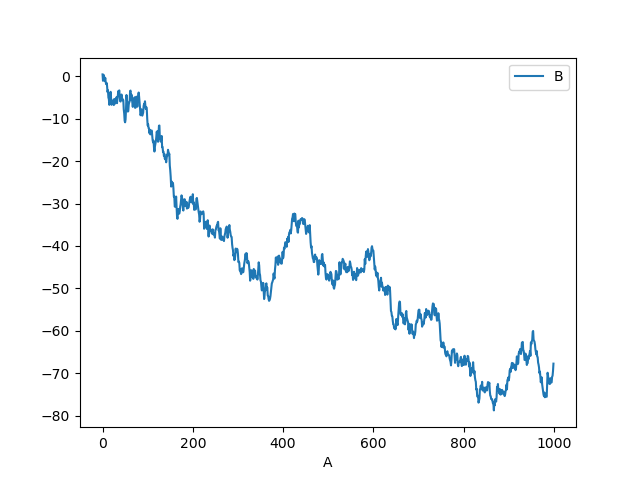Note

For more formatting and styling options, see formatting below.

## Other plots¶

Plotting methods allow for a handful of plot styles other than the default line plot. These methods can be provided as the `kind` keyword argument to `plot()`, and include:

For example, a bar plot can be created the following way:

```In : plt.figure();

In : df.iloc.plot(kind="bar");
```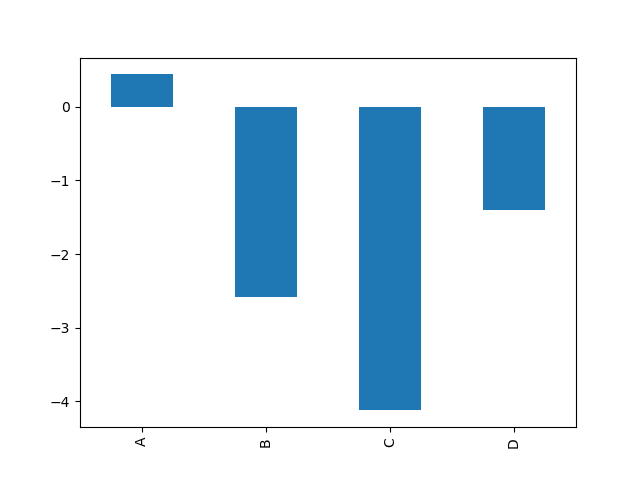You can also create these other plots using the methods `DataFrame.plot.<kind>` instead of providing the `kind` keyword argument. This makes it easier to discover plot methods and the specific arguments they use:

```In : df = pd.DataFrame()

In : df.plot.<TAB>  # noqa: E225, E999
df.plot.area     df.plot.barh     df.plot.density  df.plot.hist     df.plot.line     df.plot.scatter
df.plot.bar      df.plot.box      df.plot.hexbin   df.plot.kde      df.plot.pie
```

In addition to these `kind` s, there are the DataFrame.hist(), and DataFrame.boxplot() methods, which use a separate interface.

Finally, there are several plotting functions in `pandas.plotting` that take a `Series` or `DataFrame` as an argument. These include:

Plots may also be adorned with errorbars or tables.

### Bar plots¶

For labeled, non-time series data, you may wish to produce a bar plot:

```In : plt.figure();

In : df.iloc.plot.bar();

In : plt.axhline(0, color="k");
```Calling a DataFrame’s `plot.bar()` method produces a multiple bar plot:

```In : df2 = pd.DataFrame(np.random.rand(10, 4), columns=["a", "b", "c", "d"])

In : df2.plot.bar();
```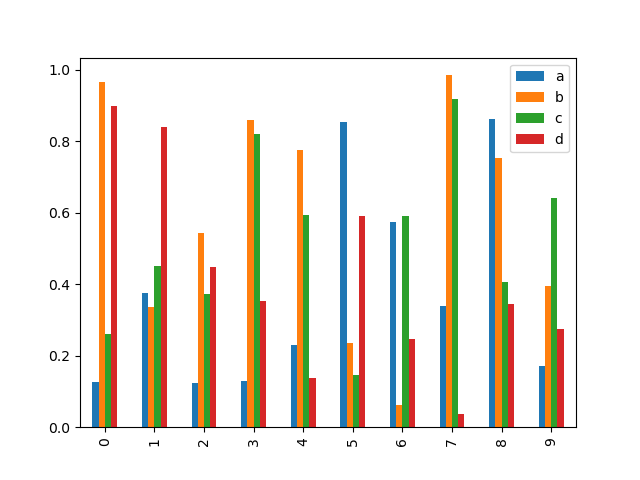To produce a stacked bar plot, pass `stacked=True`:

```In : df2.plot.bar(stacked=True);
```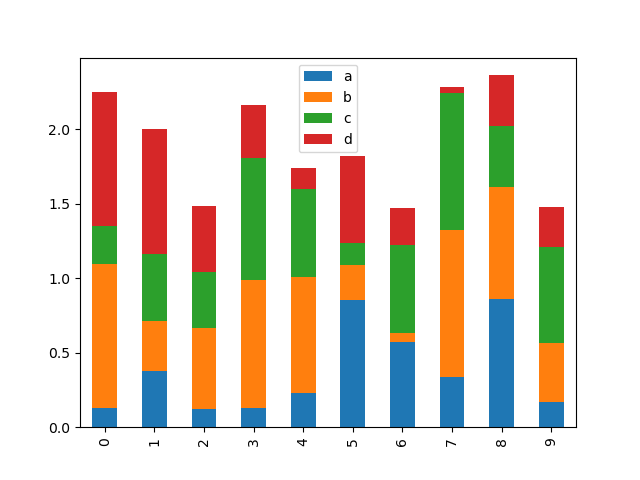To get horizontal bar plots, use the `barh` method:

```In : df2.plot.barh(stacked=True);
```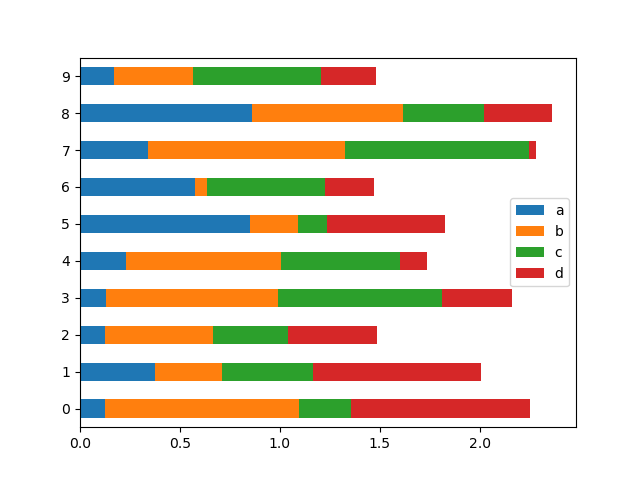### Histograms¶

Histograms can be drawn by using the `DataFrame.plot.hist()` and `Series.plot.hist()` methods.

```In : df4 = pd.DataFrame(
....:     {
....:         "a": np.random.randn(1000) + 1,
....:         "b": np.random.randn(1000),
....:         "c": np.random.randn(1000) - 1,
....:     },
....:     columns=["a", "b", "c"],
....: )
....:

In : plt.figure();

In : df4.plot.hist(alpha=0.5);
```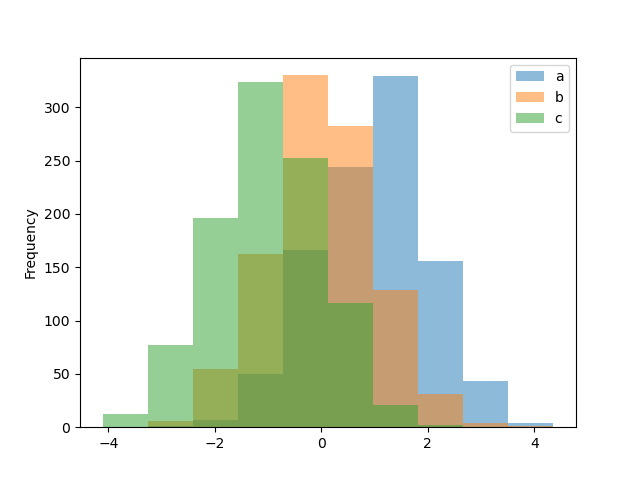A histogram can be stacked using `stacked=True`. Bin size can be changed using the `bins` keyword.

```In : plt.figure();

In : df4.plot.hist(stacked=True, bins=20);
```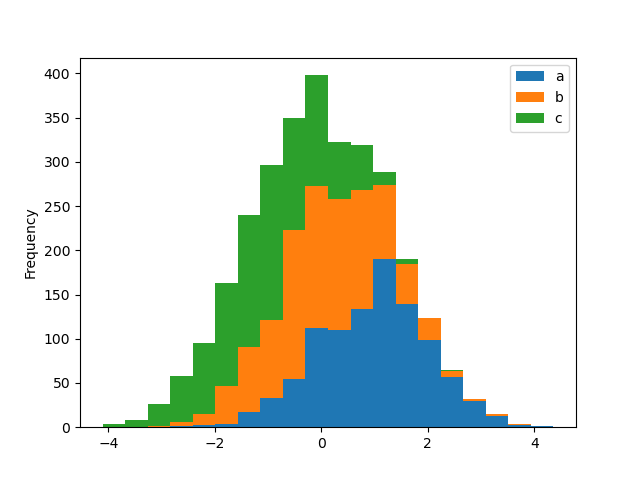You can pass other keywords supported by matplotlib `hist`. For example, horizontal and cumulative histograms can be drawn by `orientation='horizontal'` and `cumulative=True`.

```In : plt.figure();

In : df4["a"].plot.hist(orientation="horizontal", cumulative=True);
```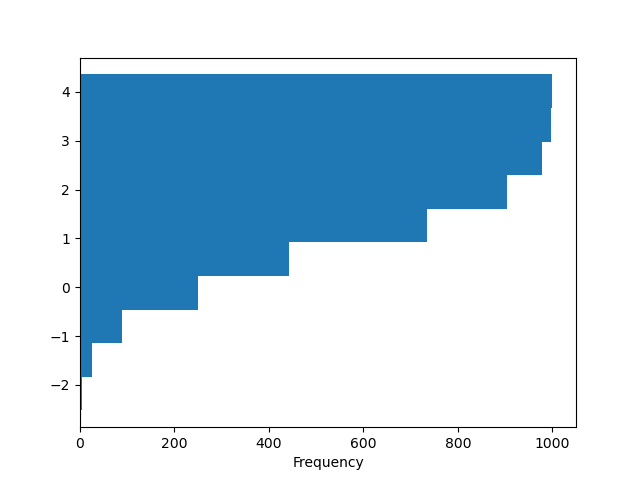See the `hist` method and the matplotlib hist documentation for more.

The existing interface `DataFrame.hist` to plot histogram still can be used.

```In : plt.figure();

In : df["A"].diff().hist();
```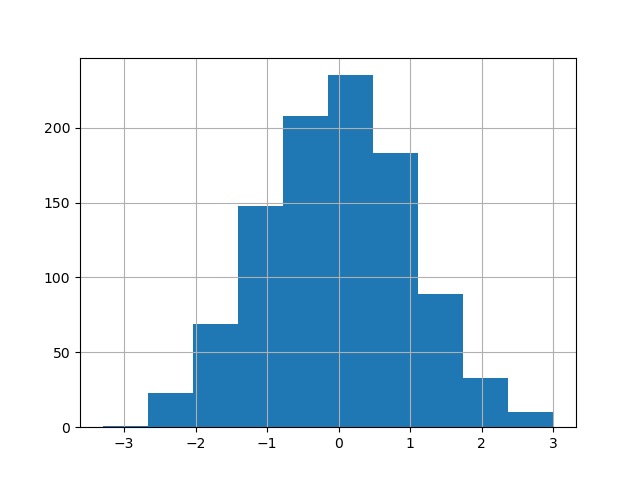`DataFrame.hist()` plots the histograms of the columns on multiple subplots:

```In : plt.figure();

In : df.diff().hist(color="k", alpha=0.5, bins=50);
```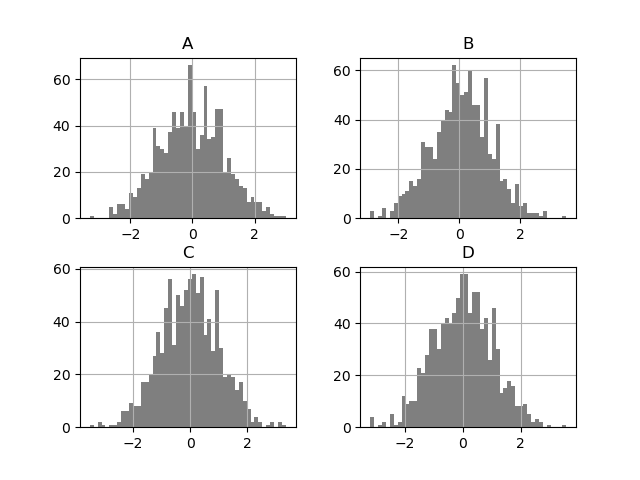The `by` keyword can be specified to plot grouped histograms:

```In : data = pd.Series(np.random.randn(1000))

In : data.hist(by=np.random.randint(0, 4, 1000), figsize=(6, 4));
```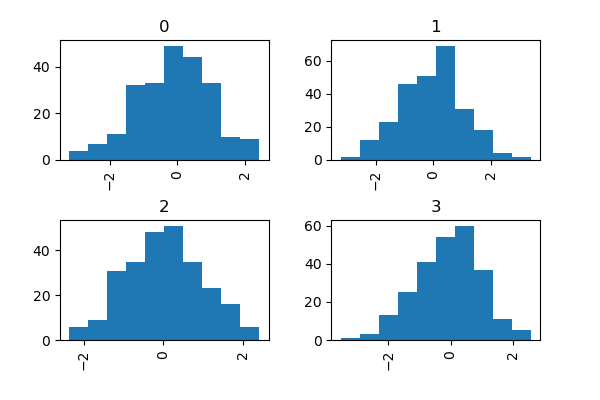### Box plots¶

Boxplot can be drawn calling `Series.plot.box()` and `DataFrame.plot.box()`, or `DataFrame.boxplot()` to visualize the distribution of values within each column.

For instance, here is a boxplot representing five trials of 10 observations of a uniform random variable on [0,1).

```In : df = pd.DataFrame(np.random.rand(10, 5), columns=["A", "B", "C", "D", "E"])

In : df.plot.box();
```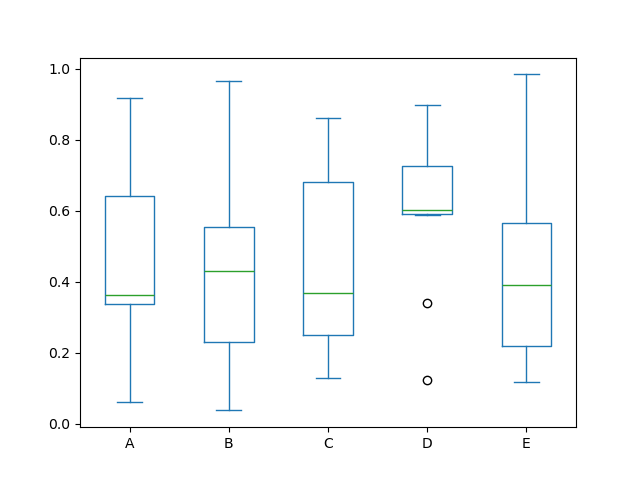Boxplot can be colorized by passing `color` keyword. You can pass a `dict` whose keys are `boxes`, `whiskers`, `medians` and `caps`. If some keys are missing in the `dict`, default colors are used for the corresponding artists. Also, boxplot has `sym` keyword to specify fliers style.

When you pass other type of arguments via `color` keyword, it will be directly passed to matplotlib for all the `boxes`, `whiskers`, `medians` and `caps` colorization.

The colors are applied to every boxes to be drawn. If you want more complicated colorization, you can get each drawn artists by passing return_type.

```In : color = {
....:     "boxes": "DarkGreen",
....:     "whiskers": "DarkOrange",
....:     "medians": "DarkBlue",
....:     "caps": "Gray",
....: }
....:

In : df.plot.box(color=color, sym="r+");
```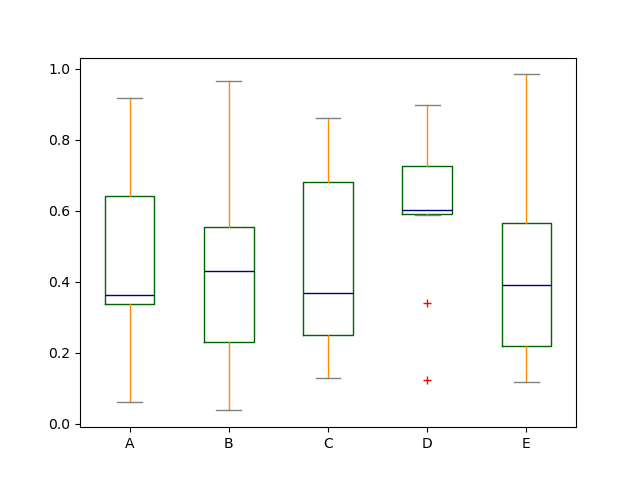Also, you can pass other keywords supported by matplotlib `boxplot`. For example, horizontal and custom-positioned boxplot can be drawn by `vert=False` and `positions` keywords.

```In : df.plot.box(vert=False, positions=[1, 4, 5, 6, 8]);
```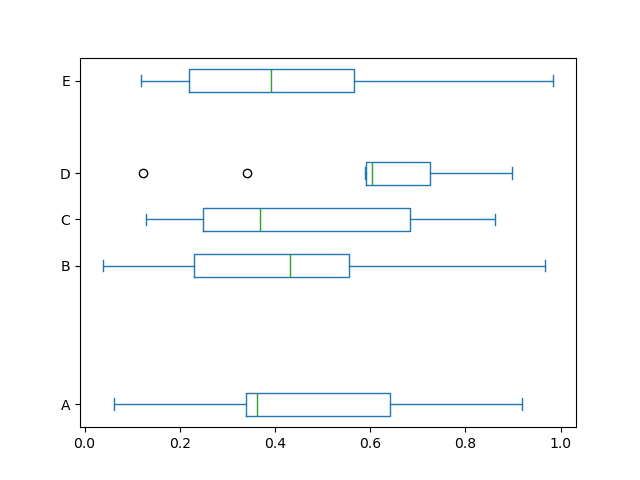See the `boxplot` method and the matplotlib boxplot documentation for more.

The existing interface `DataFrame.boxplot` to plot boxplot still can be used.

```In : df = pd.DataFrame(np.random.rand(10, 5))

In : plt.figure();

In : bp = df.boxplot()
```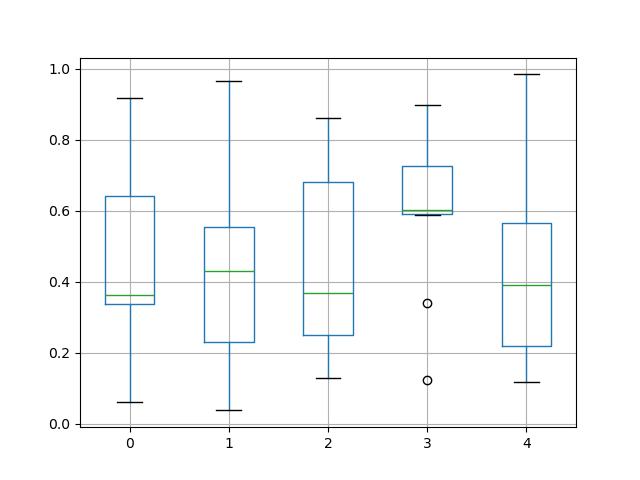You can create a stratified boxplot using the `by` keyword argument to create groupings. For instance,

```In : df = pd.DataFrame(np.random.rand(10, 2), columns=["Col1", "Col2"])

In : df["X"] = pd.Series(["A", "A", "A", "A", "A", "B", "B", "B", "B", "B"])

In : plt.figure();

In : bp = df.boxplot(by="X")
```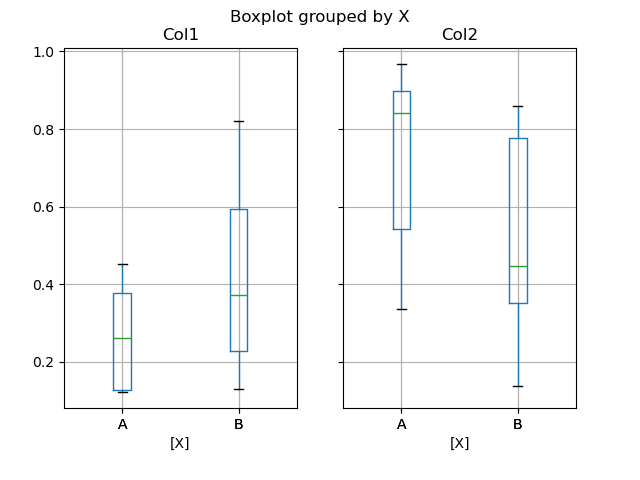You can also pass a subset of columns to plot, as well as group by multiple columns:

```In : df = pd.DataFrame(np.random.rand(10, 3), columns=["Col1", "Col2", "Col3"])

In : df["X"] = pd.Series(["A", "A", "A", "A", "A", "B", "B", "B", "B", "B"])

In : df["Y"] = pd.Series(["A", "B", "A", "B", "A", "B", "A", "B", "A", "B"])

In : plt.figure();

In : bp = df.boxplot(column=["Col1", "Col2"], by=["X", "Y"])
```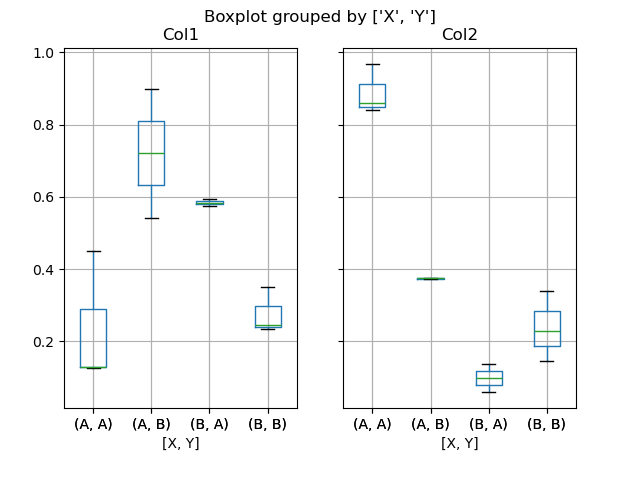In `boxplot`, the return type can be controlled by the `return_type`, keyword. The valid choices are `{"axes", "dict", "both", None}`. Faceting, created by `DataFrame.boxplot` with the `by` keyword, will affect the output type as well:

`return_type`

Faceted

Output type

`None`

No

axes

`None`

Yes

2-D ndarray of axes

`'axes'`

No

axes

`'axes'`

Yes

Series of axes

`'dict'`

No

dict of artists

`'dict'`

Yes

Series of dicts of artists

`'both'`

No

namedtuple

`'both'`

Yes

Series of namedtuples

`Groupby.boxplot` always returns a `Series` of `return_type`.

```In : np.random.seed(1234)

In : df_box = pd.DataFrame(np.random.randn(50, 2))

In : df_box["g"] = np.random.choice(["A", "B"], size=50)

In : df_box.loc[df_box["g"] == "B", 1] += 3

In : bp = df_box.boxplot(by="g")
```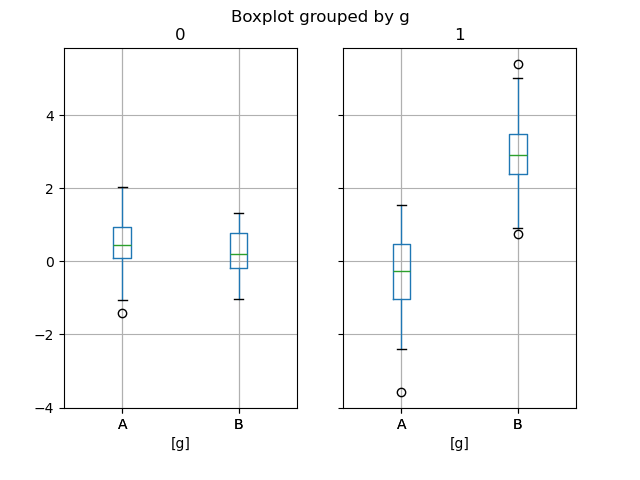The subplots above are split by the numeric columns first, then the value of the `g` column. Below the subplots are first split by the value of `g`, then by the numeric columns.

```In : bp = df_box.groupby("g").boxplot()
```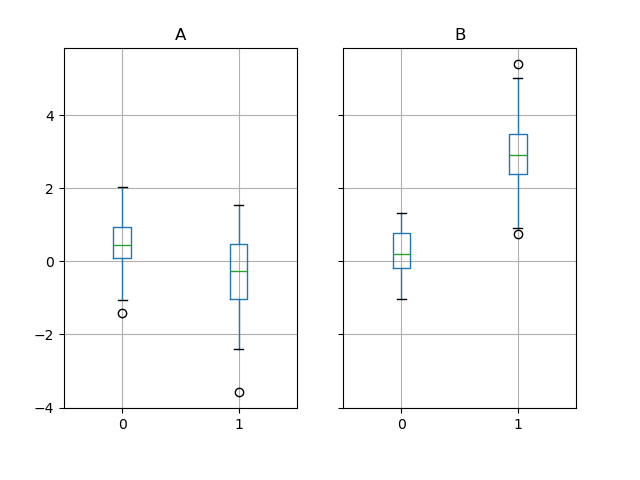### Area plot¶

You can create area plots with `Series.plot.area()` and `DataFrame.plot.area()`. Area plots are stacked by default. To produce stacked area plot, each column must be either all positive or all negative values.

When input data contains `NaN`, it will be automatically filled by 0. If you want to drop or fill by different values, use `dataframe.dropna()` or `dataframe.fillna()` before calling `plot`.

```In : df = pd.DataFrame(np.random.rand(10, 4), columns=["a", "b", "c", "d"])

In : df.plot.area();
```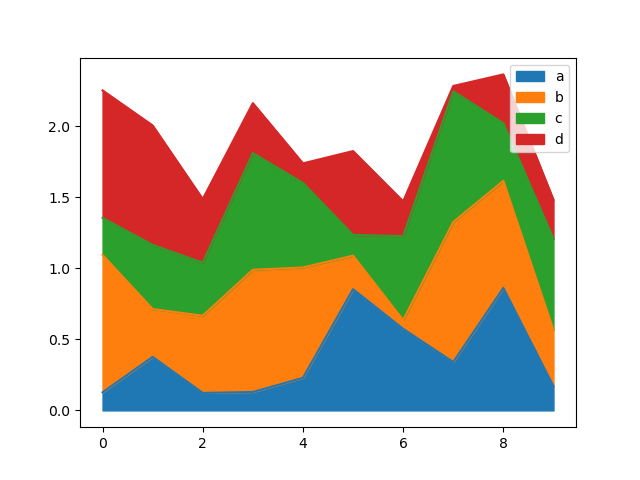To produce an unstacked plot, pass `stacked=False`. Alpha value is set to 0.5 unless otherwise specified:

```In : df.plot.area(stacked=False);
```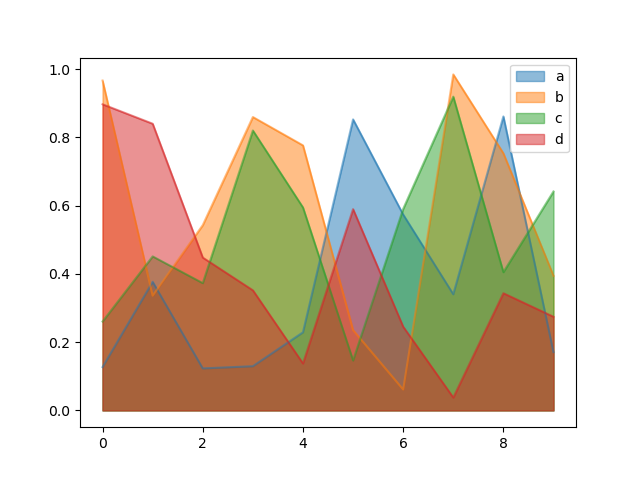### Scatter plot¶

Scatter plot can be drawn by using the `DataFrame.plot.scatter()` method. Scatter plot requires numeric columns for the x and y axes. These can be specified by the `x` and `y` keywords.

```In : df = pd.DataFrame(np.random.rand(50, 4), columns=["a", "b", "c", "d"])

In : df["species"] = pd.Categorical(
....:     ["setosa"] * 20 + ["versicolor"] * 20 + ["virginica"] * 10
....: )
....:

In : df.plot.scatter(x="a", y="b");
```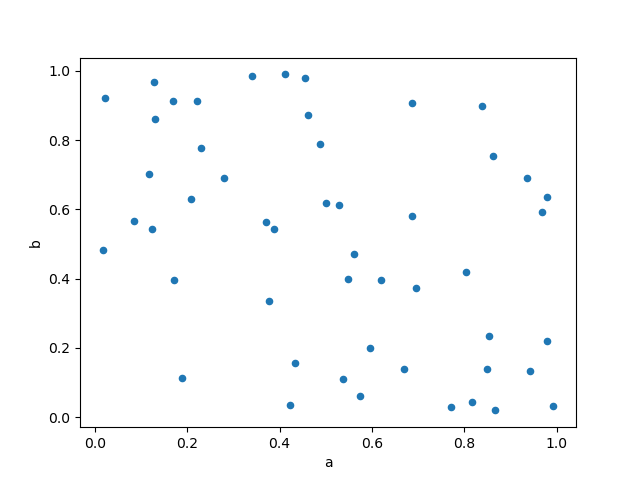To plot multiple column groups in a single axes, repeat `plot` method specifying target `ax`. It is recommended to specify `color` and `label` keywords to distinguish each groups.

```In : ax = df.plot.scatter(x="a", y="b", color="DarkBlue", label="Group 1")

In : df.plot.scatter(x="c", y="d", color="DarkGreen", label="Group 2", ax=ax);
```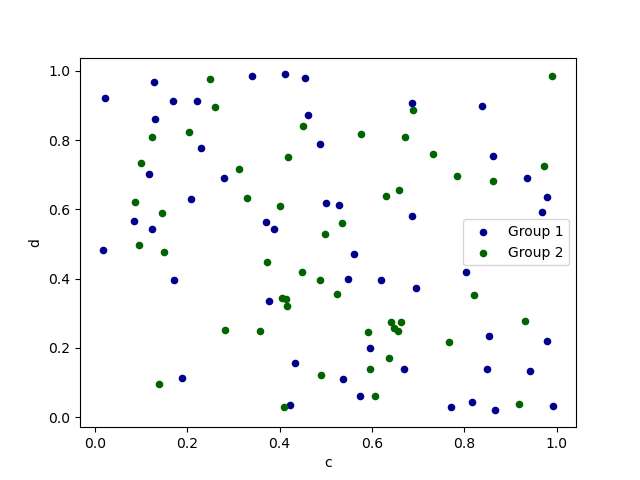The keyword `c` may be given as the name of a column to provide colors for each point:

```In : df.plot.scatter(x="a", y="b", c="c", s=50);
```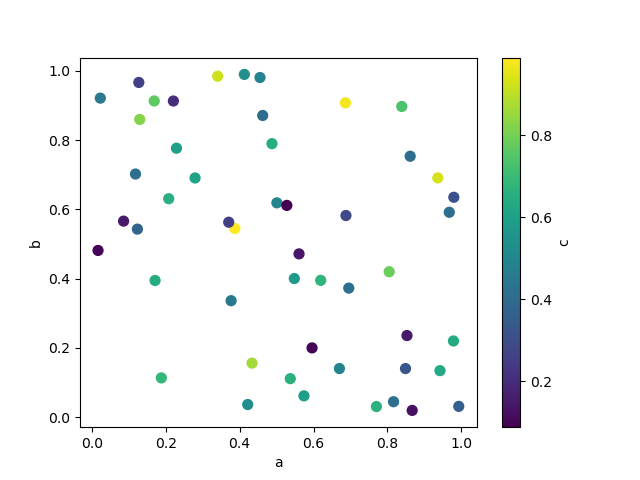If a categorical column is passed to `c`, then a discrete colorbar will be produced:

New in version 1.3.0.

```In : df.plot.scatter(x="a", y="b", c="species", cmap="viridis", s=50);
```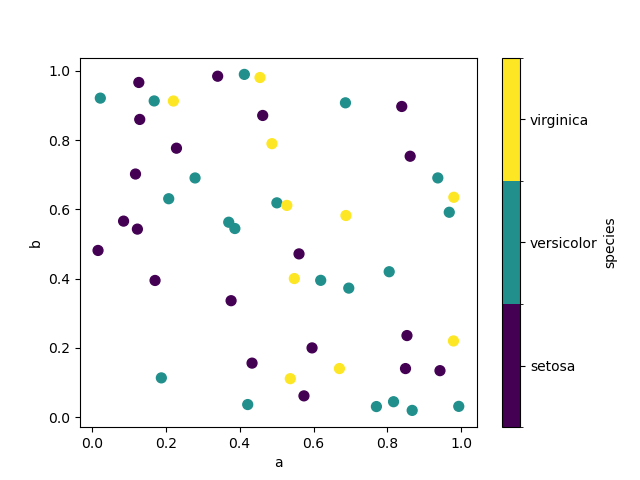You can pass other keywords supported by matplotlib `scatter`. The example below shows a bubble chart using a column of the `DataFrame` as the bubble size.

```In : df.plot.scatter(x="a", y="b", s=df["c"] * 200);
```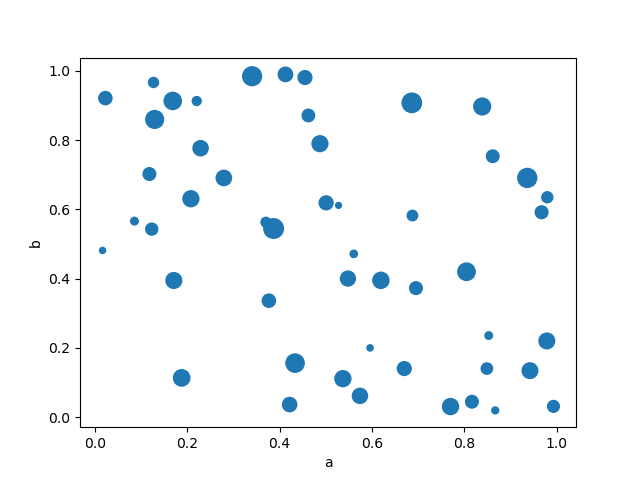See the `scatter` method and the matplotlib scatter documentation for more.

### Hexagonal bin plot¶

You can create hexagonal bin plots with `DataFrame.plot.hexbin()`. Hexbin plots can be a useful alternative to scatter plots if your data are too dense to plot each point individually.

```In : df = pd.DataFrame(np.random.randn(1000, 2), columns=["a", "b"])

In : df["b"] = df["b"] + np.arange(1000)

In : df.plot.hexbin(x="a", y="b", gridsize=25);
```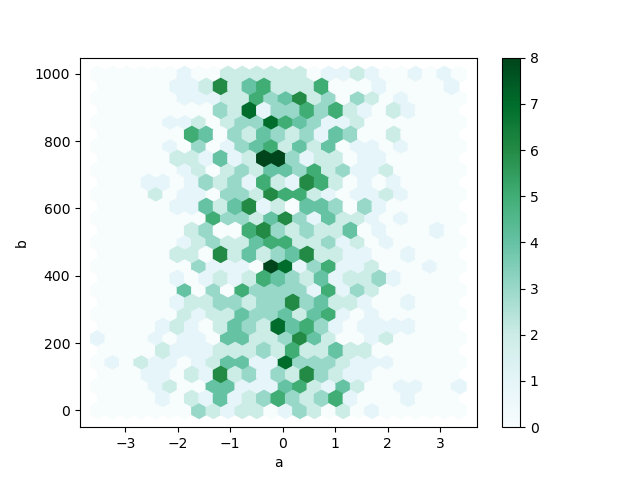A useful keyword argument is `gridsize`; it controls the number of hexagons in the x-direction, and defaults to 100. A larger `gridsize` means more, smaller bins.

By default, a histogram of the counts around each `(x, y)` point is computed. You can specify alternative aggregations by passing values to the `C` and `reduce_C_function` arguments. `C` specifies the value at each `(x, y)` point and `reduce_C_function` is a function of one argument that reduces all the values in a bin to a single number (e.g. `mean`, `max`, `sum`, `std`). In this example the positions are given by columns `a` and `b`, while the value is given by column `z`. The bins are aggregated with NumPy’s `max` function.

```In : df = pd.DataFrame(np.random.randn(1000, 2), columns=["a", "b"])

In : df["b"] = df["b"] + np.arange(1000)

In : df["z"] = np.random.uniform(0, 3, 1000)

In : df.plot.hexbin(x="a", y="b", C="z", reduce_C_function=np.max, gridsize=25);
```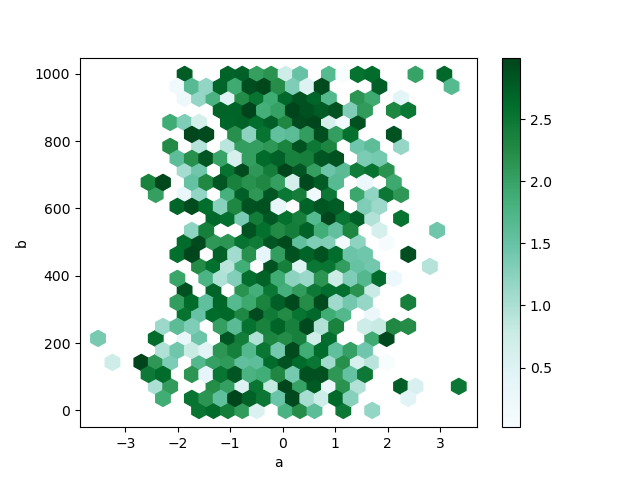See the `hexbin` method and the matplotlib hexbin documentation for more.

### Pie plot¶

You can create a pie plot with `DataFrame.plot.pie()` or `Series.plot.pie()`. If your data includes any `NaN`, they will be automatically filled with 0. A `ValueError` will be raised if there are any negative values in your data.

```In : series = pd.Series(3 * np.random.rand(4), index=["a", "b", "c", "d"], name="series")

In : series.plot.pie(figsize=(6, 6));
```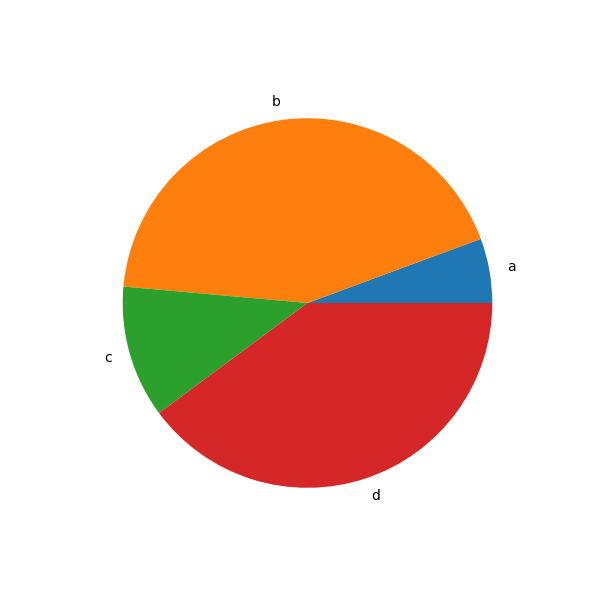For pie plots it’s best to use square figures, i.e. a figure aspect ratio 1. You can create the figure with equal width and height, or force the aspect ratio to be equal after plotting by calling `ax.set_aspect('equal')` on the returned `axes` object.

Note that pie plot with `DataFrame` requires that you either specify a target column by the `y` argument or `subplots=True`. When `y` is specified, pie plot of selected column will be drawn. If `subplots=True` is specified, pie plots for each column are drawn as subplots. A legend will be drawn in each pie plots by default; specify `legend=False` to hide it.

```In : df = pd.DataFrame(
....:     3 * np.random.rand(4, 2), index=["a", "b", "c", "d"], columns=["x", "y"]
....: )
....:

In : df.plot.pie(subplots=True, figsize=(8, 4));
```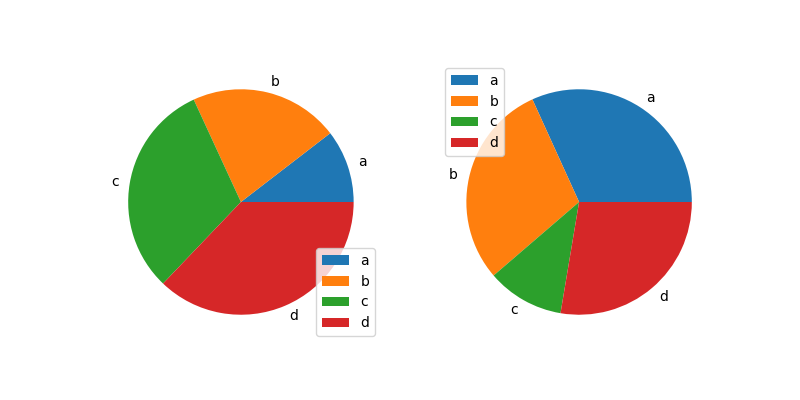You can use the `labels` and `colors` keywords to specify the labels and colors of each wedge.

Warning

Most pandas plots use the `label` and `color` arguments (note the lack of “s” on those). To be consistent with `matplotlib.pyplot.pie()` you must use `labels` and `colors`.

If you want to hide wedge labels, specify `labels=None`. If `fontsize` is specified, the value will be applied to wedge labels. Also, other keywords supported by `matplotlib.pyplot.pie()` can be used.

```In : series.plot.pie(
....:     labels=["AA", "BB", "CC", "DD"],
....:     colors=["r", "g", "b", "c"],
....:     autopct="%.2f",
....:     fontsize=20,
....:     figsize=(6, 6),
....: );
....:
```If you pass values whose sum total is less than 1.0, matplotlib draws a semicircle.

```In : series = pd.Series([0.1] * 4, index=["a", "b", "c", "d"], name="series2")

In : series.plot.pie(figsize=(6, 6));
```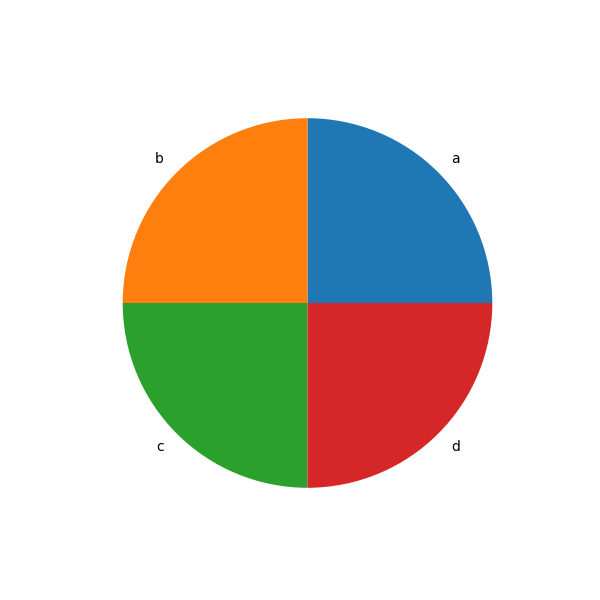See the matplotlib pie documentation for more.

## Plotting with missing data¶

pandas tries to be pragmatic about plotting `DataFrames` or `Series` that contain missing data. Missing values are dropped, left out, or filled depending on the plot type.

Plot Type

NaN Handling

Line

Leave gaps at NaNs

Line (stacked)

Fill 0’s

Bar

Fill 0’s

Scatter

Drop NaNs

Histogram

Drop NaNs (column-wise)

Box

Drop NaNs (column-wise)

Area

Fill 0’s

KDE

Drop NaNs (column-wise)

Hexbin

Drop NaNs

Pie

Fill 0’s

If any of these defaults are not what you want, or if you want to be explicit about how missing values are handled, consider using `fillna()` or `dropna()` before plotting.

## Plotting tools¶

These functions can be imported from `pandas.plotting` and take a `Series` or `DataFrame` as an argument.

### Scatter matrix plot¶

You can create a scatter plot matrix using the `scatter_matrix` method in `pandas.plotting`:

```In : from pandas.plotting import scatter_matrix

In : df = pd.DataFrame(np.random.randn(1000, 4), columns=["a", "b", "c", "d"])

In : scatter_matrix(df, alpha=0.2, figsize=(6, 6), diagonal="kde");
```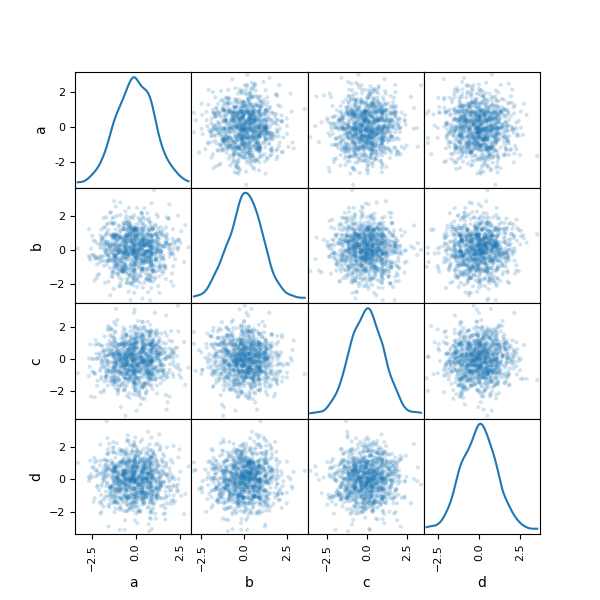### Density plot¶

You can create density plots using the `Series.plot.kde()` and `DataFrame.plot.kde()` methods.

```In : ser = pd.Series(np.random.randn(1000))

In : ser.plot.kde();
```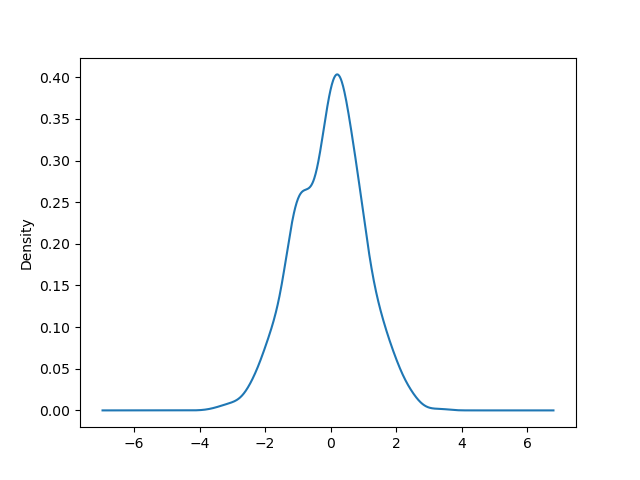### Andrews curves¶

Andrews curves allow one to plot multivariate data as a large number of curves that are created using the attributes of samples as coefficients for Fourier series, see the Wikipedia entry for more information. By coloring these curves differently for each class it is possible to visualize data clustering. Curves belonging to samples of the same class will usually be closer together and form larger structures.

Note: The “Iris” dataset is available here.

```In : from pandas.plotting import andrews_curves

In : plt.figure();

In : andrews_curves(data, "Name");
```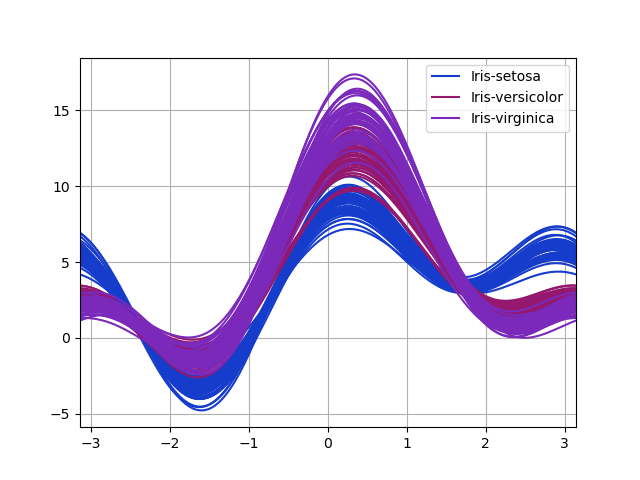### Parallel coordinates¶

Parallel coordinates is a plotting technique for plotting multivariate data, see the Wikipedia entry for an introduction. Parallel coordinates allows one to see clusters in data and to estimate other statistics visually. Using parallel coordinates points are represented as connected line segments. Each vertical line represents one attribute. One set of connected line segments represents one data point. Points that tend to cluster will appear closer together.

```In : from pandas.plotting import parallel_coordinates

In : plt.figure();

In : parallel_coordinates(data, "Name");
```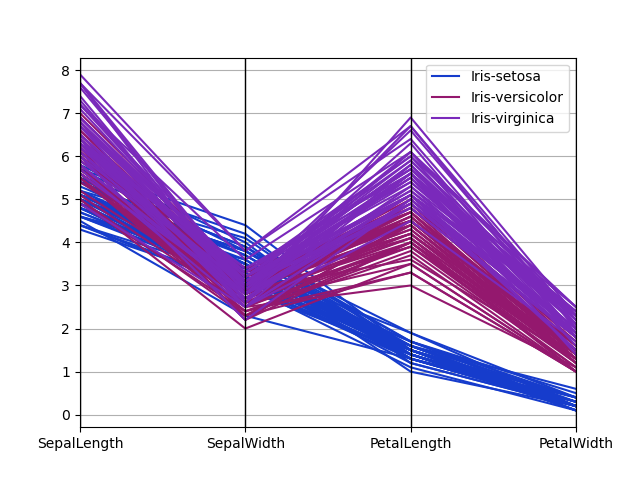### Lag plot¶

Lag plots are used to check if a data set or time series is random. Random data should not exhibit any structure in the lag plot. Non-random structure implies that the underlying data are not random. The `lag` argument may be passed, and when `lag=1` the plot is essentially `data[:-1]` vs. `data[1:]`.

```In : from pandas.plotting import lag_plot

In : plt.figure();

In : spacing = np.linspace(-99 * np.pi, 99 * np.pi, num=1000)

In : data = pd.Series(0.1 * np.random.rand(1000) + 0.9 * np.sin(spacing))

In : lag_plot(data);
```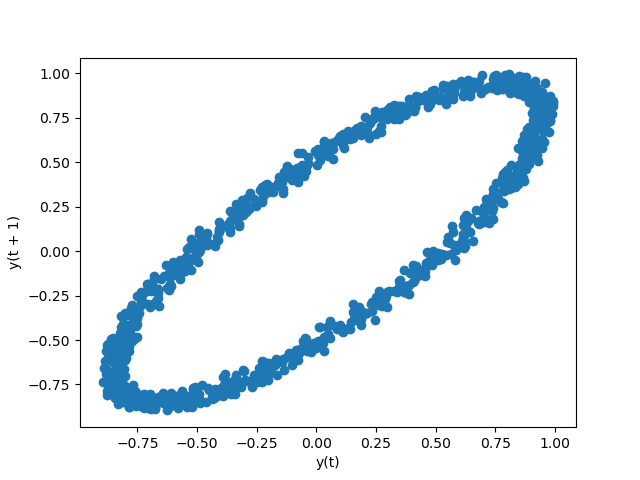### Autocorrelation plot¶

Autocorrelation plots are often used for checking randomness in time series. This is done by computing autocorrelations for data values at varying time lags. If time series is random, such autocorrelations should be near zero for any and all time-lag separations. If time series is non-random then one or more of the autocorrelations will be significantly non-zero. The horizontal lines displayed in the plot correspond to 95% and 99% confidence bands. The dashed line is 99% confidence band. See the Wikipedia entry for more about autocorrelation plots.

```In : from pandas.plotting import autocorrelation_plot

In : plt.figure();

In : spacing = np.linspace(-9 * np.pi, 9 * np.pi, num=1000)

In : data = pd.Series(0.7 * np.random.rand(1000) + 0.3 * np.sin(spacing))

In : autocorrelation_plot(data);
```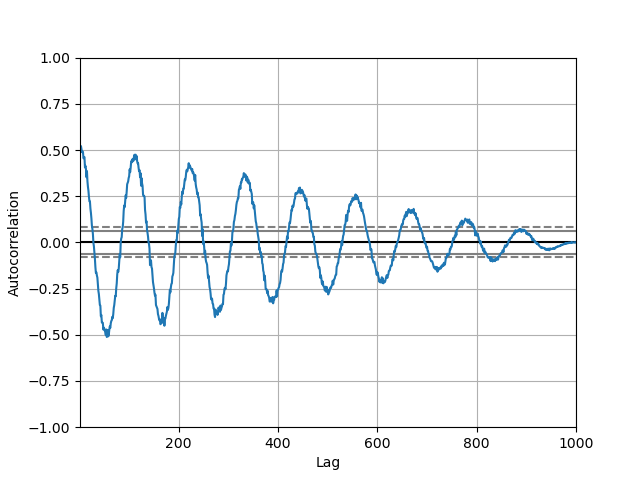### Bootstrap plot¶

Bootstrap plots are used to visually assess the uncertainty of a statistic, such as mean, median, midrange, etc. A random subset of a specified size is selected from a data set, the statistic in question is computed for this subset and the process is repeated a specified number of times. Resulting plots and histograms are what constitutes the bootstrap plot.

```In : from pandas.plotting import bootstrap_plot

In : data = pd.Series(np.random.rand(1000))

In : bootstrap_plot(data, size=50, samples=500, color="grey");
```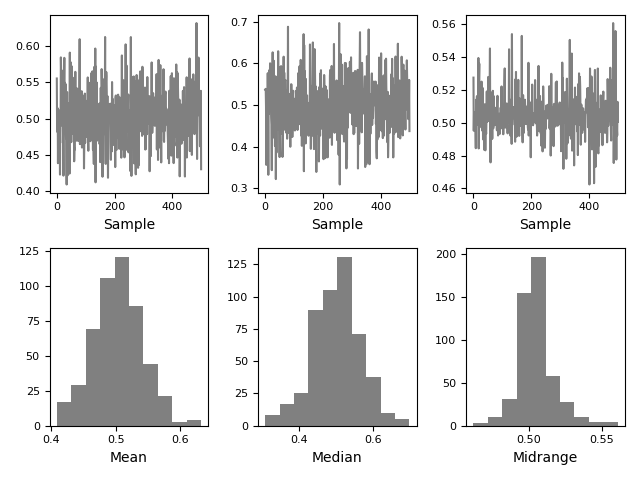RadViz is a way of visualizing multi-variate data. It is based on a simple spring tension minimization algorithm. Basically you set up a bunch of points in a plane. In our case they are equally spaced on a unit circle. Each point represents a single attribute. You then pretend that each sample in the data set is attached to each of these points by a spring, the stiffness of which is proportional to the numerical value of that attribute (they are normalized to unit interval). The point in the plane, where our sample settles to (where the forces acting on our sample are at an equilibrium) is where a dot representing our sample will be drawn. Depending on which class that sample belongs it will be colored differently. See the R package Radviz for more information.

Note: The “Iris” dataset is available here.

```In : from pandas.plotting import radviz

In : plt.figure();

```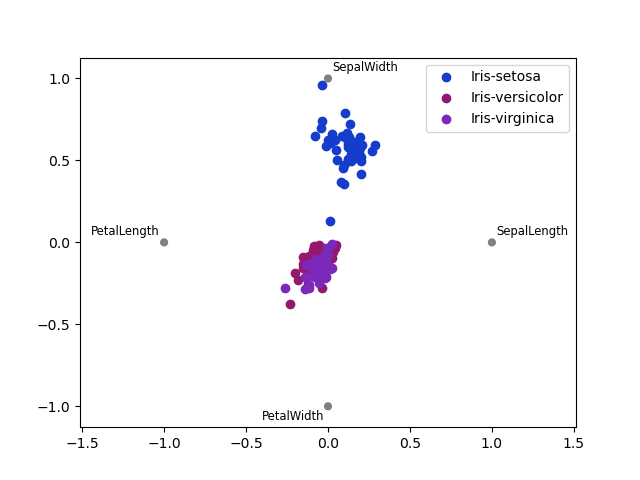## Plot formatting¶

### Setting the plot style¶

From version 1.5 and up, matplotlib offers a range of pre-configured plotting styles. Setting the style can be used to easily give plots the general look that you want. Setting the style is as easy as calling `matplotlib.style.use(my_plot_style)` before creating your plot. For example you could write `matplotlib.style.use('ggplot')` for ggplot-style plots.

You can see the various available style names at `matplotlib.style.available` and it’s very easy to try them out.

### General plot style arguments¶

Most plotting methods have a set of keyword arguments that control the layout and formatting of the returned plot:

```In : plt.figure();

In : ts.plot(style="k--", label="Series");
```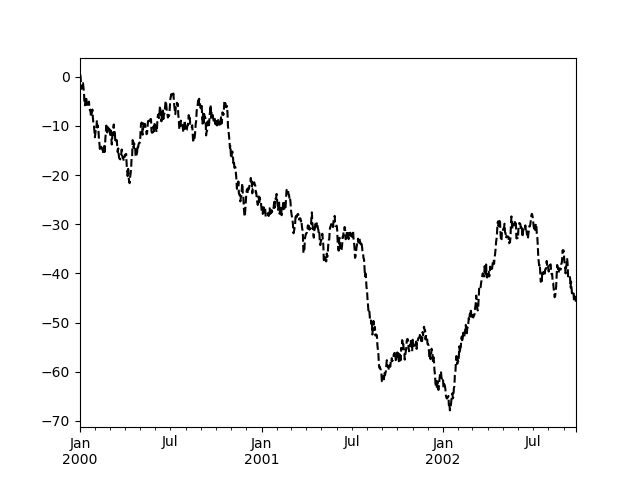For each kind of plot (e.g. `line`, `bar`, `scatter`) any additional arguments keywords are passed along to the corresponding matplotlib function (`ax.plot()`, `ax.bar()`, `ax.scatter()`). These can be used to control additional styling, beyond what pandas provides.

### Controlling the legend¶

You may set the `legend` argument to `False` to hide the legend, which is shown by default.

```In : df = pd.DataFrame(np.random.randn(1000, 4), index=ts.index, columns=list("ABCD"))

In : df = df.cumsum()

In : df.plot(legend=False);
```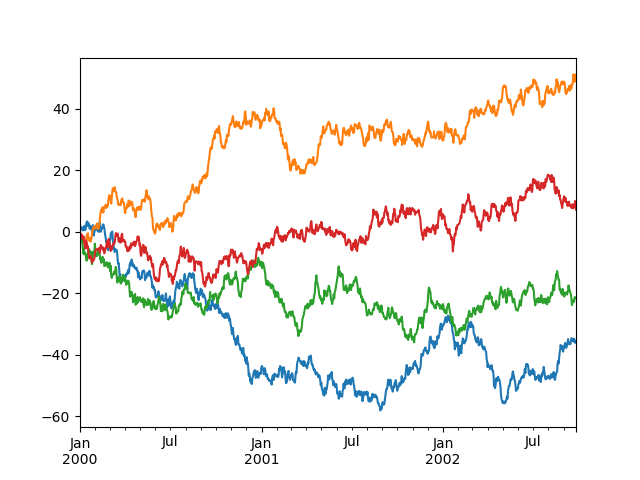### Controlling the labels¶

New in version 1.1.0.

You may set the `xlabel` and `ylabel` arguments to give the plot custom labels for x and y axis. By default, pandas will pick up index name as xlabel, while leaving it empty for ylabel.

```
```
```In : df.plot();

In : df.plot(xlabel="new x", ylabel="new y");
```### Scales¶

You may pass `logy` to get a log-scale Y axis.

```In : ts = pd.Series(np.random.randn(1000), index=pd.date_range("1/1/2000", periods=1000))

In : ts = np.exp(ts.cumsum())

In : ts.plot(logy=True);
```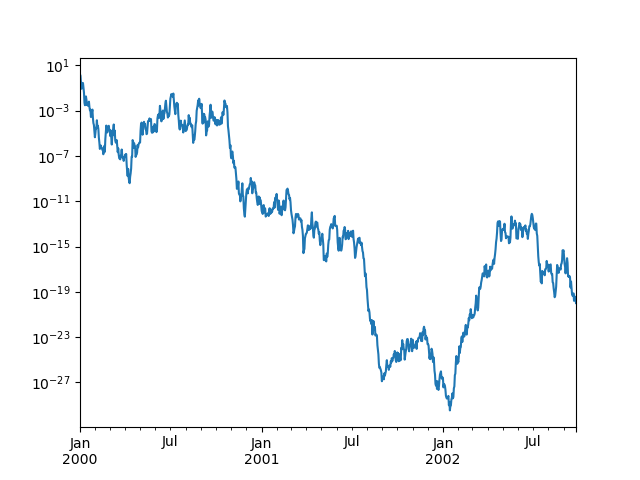See also the `logx` and `loglog` keyword arguments.

### Plotting on a secondary y-axis¶

To plot data on a secondary y-axis, use the `secondary_y` keyword:

```In : df["A"].plot();

In : df["B"].plot(secondary_y=True, style="g");
```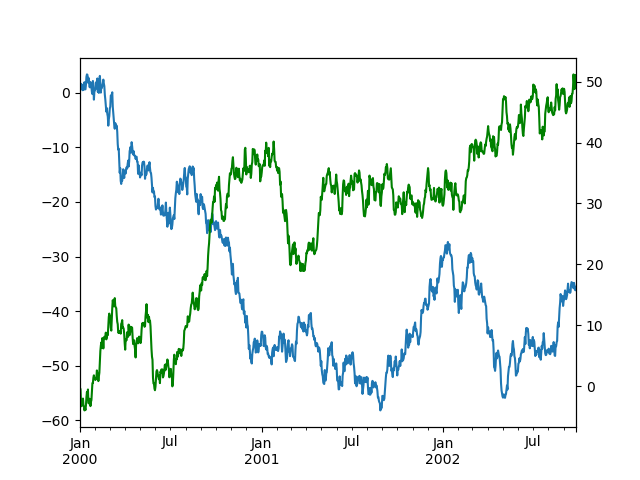To plot some columns in a `DataFrame`, give the column names to the `secondary_y` keyword:

```In : plt.figure();

In : ax = df.plot(secondary_y=["A", "B"])

In : ax.set_ylabel("CD scale");

In : ax.right_ax.set_ylabel("AB scale");
```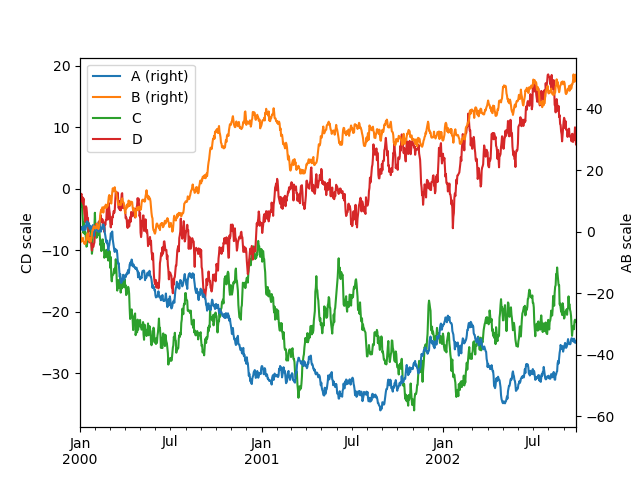Note that the columns plotted on the secondary y-axis is automatically marked with “(right)” in the legend. To turn off the automatic marking, use the `mark_right=False` keyword:

```In : plt.figure();

In : df.plot(secondary_y=["A", "B"], mark_right=False);
```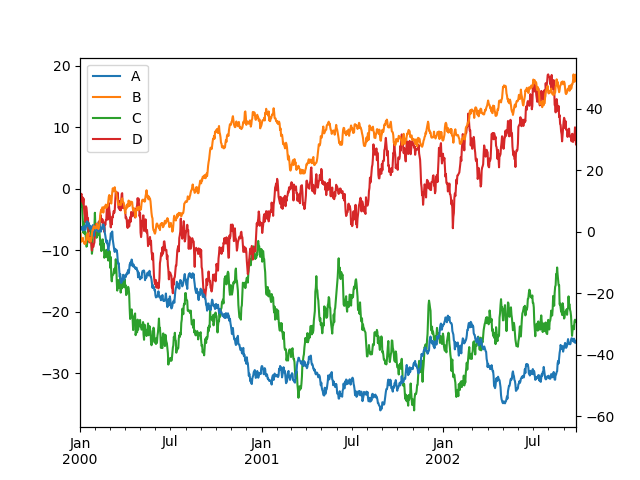### Custom formatters for timeseries plots¶

Changed in version 1.0.0.

pandas provides custom formatters for timeseries plots. These change the formatting of the axis labels for dates and times. By default, the custom formatters are applied only to plots created by pandas with `DataFrame.plot()` or `Series.plot()`. To have them apply to all plots, including those made by matplotlib, set the option `pd.options.plotting.matplotlib.register_converters = True` or use `pandas.plotting.register_matplotlib_converters()`.

pandas includes automatic tick resolution adjustment for regular frequency time-series data. For limited cases where pandas cannot infer the frequency information (e.g., in an externally created `twinx`), you can choose to suppress this behavior for alignment purposes.

Here is the default behavior, notice how the x-axis tick labeling is performed:

```In : plt.figure();

In : df["A"].plot();
```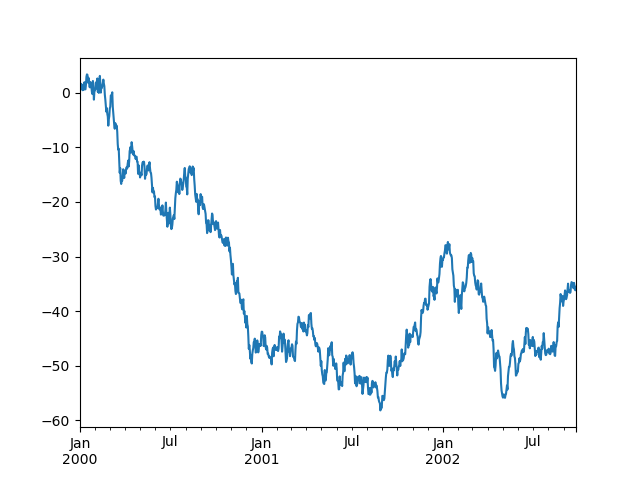Using the `x_compat` parameter, you can suppress this behavior:

```In : plt.figure();

In : df["A"].plot(x_compat=True);
```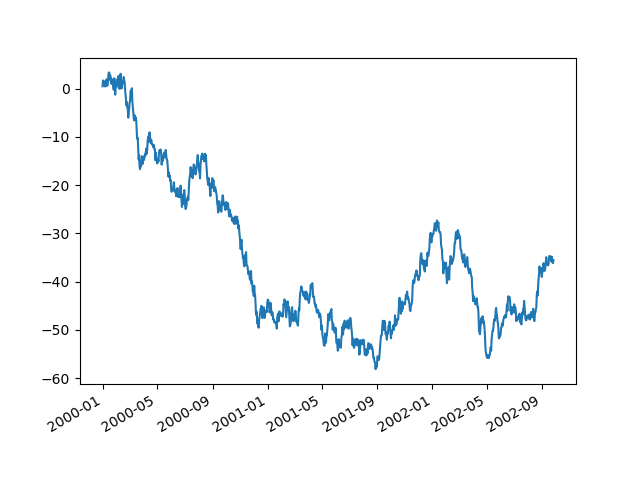If you have more than one plot that needs to be suppressed, the `use` method in `pandas.plotting.plot_params` can be used in a `with` statement:

```In : plt.figure();

In : with pd.plotting.plot_params.use("x_compat", True):
.....:     df["A"].plot(color="r")
.....:     df["B"].plot(color="g")
.....:     df["C"].plot(color="b")
.....:
```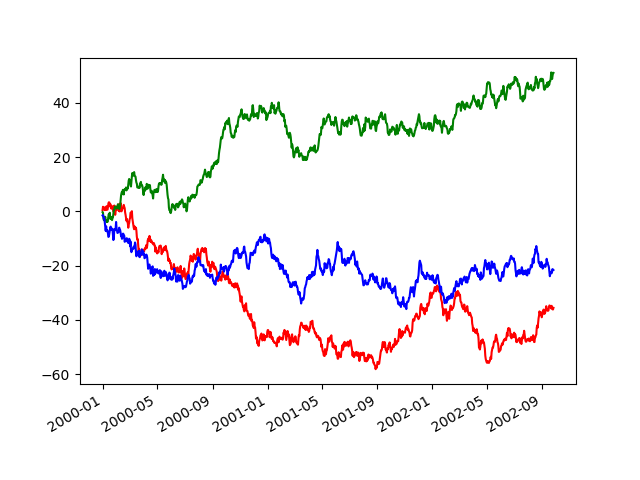`TimedeltaIndex` now uses the native matplotlib tick locator methods, it is useful to call the automatic date tick adjustment from matplotlib for figures whose ticklabels overlap.

See the `autofmt_xdate` method and the matplotlib documentation for more.

### Subplots¶

Each `Series` in a `DataFrame` can be plotted on a different axis with the `subplots` keyword:

```In : df.plot(subplots=True, figsize=(6, 6));
```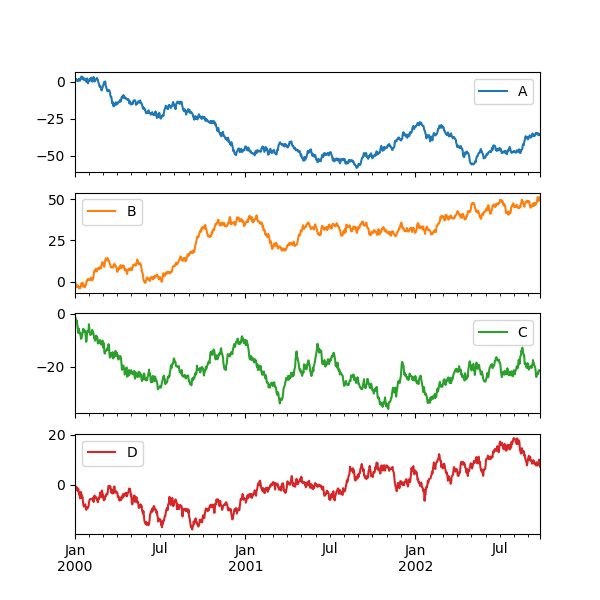### Using layout and targeting multiple axes¶

The layout of subplots can be specified by the `layout` keyword. It can accept `(rows, columns)`. The `layout` keyword can be used in `hist` and `boxplot` also. If the input is invalid, a `ValueError` will be raised.

The number of axes which can be contained by rows x columns specified by `layout` must be larger than the number of required subplots. If layout can contain more axes than required, blank axes are not drawn. Similar to a NumPy array’s `reshape` method, you can use `-1` for one dimension to automatically calculate the number of rows or columns needed, given the other.

```In : df.plot(subplots=True, layout=(2, 3), figsize=(6, 6), sharex=False);
```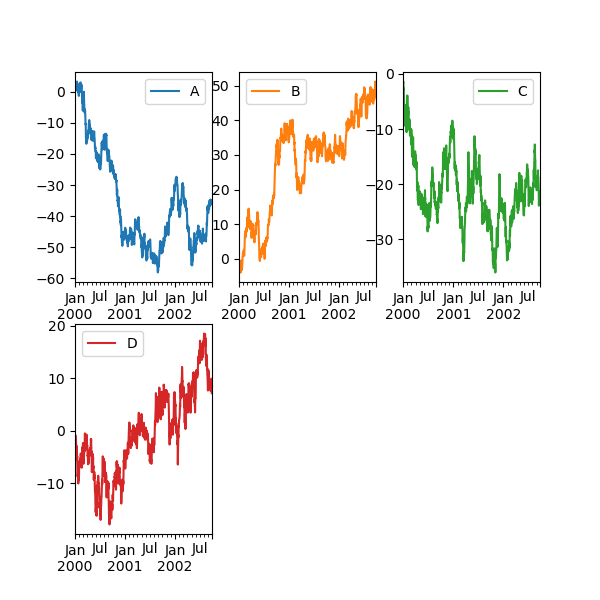The above example is identical to using:

```In : df.plot(subplots=True, layout=(2, -1), figsize=(6, 6), sharex=False);
```

The required number of columns (3) is inferred from the number of series to plot and the given number of rows (2).

You can pass multiple axes created beforehand as list-like via `ax` keyword. This allows more complicated layouts. The passed axes must be the same number as the subplots being drawn.

When multiple axes are passed via the `ax` keyword, `layout`, `sharex` and `sharey` keywords don’t affect to the output. You should explicitly pass `sharex=False` and `sharey=False`, otherwise you will see a warning.

```In : fig, axes = plt.subplots(4, 4, figsize=(9, 9))

In : target1 = [axes, axes, axes, axes]

In : target2 = [axes, axes, axes, axes]

In : df.plot(subplots=True, ax=target1, legend=False, sharex=False, sharey=False);

In : (-df).plot(subplots=True, ax=target2, legend=False, sharex=False, sharey=False);
```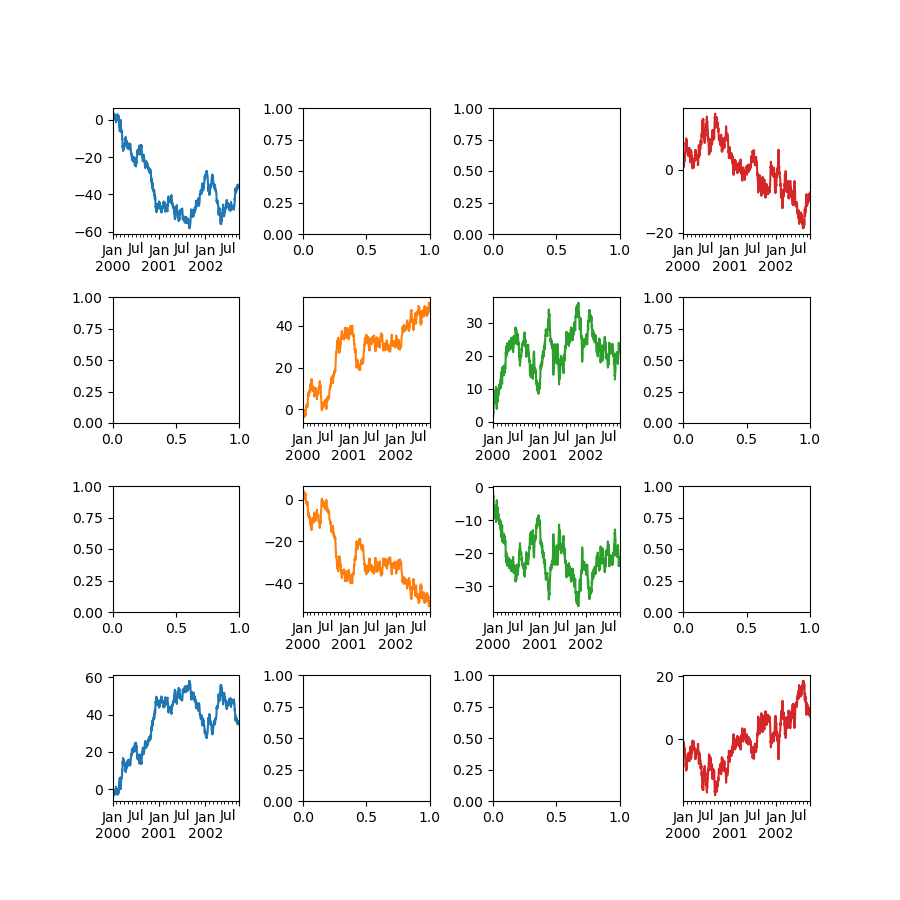Another option is passing an `ax` argument to `Series.plot()` to plot on a particular axis:

```In : fig, axes = plt.subplots(nrows=2, ncols=2)

In : df["A"].plot(ax=axes[0, 0]);

In : axes[0, 0].set_title("A");

In : df["B"].plot(ax=axes[0, 1]);

In : axes[0, 1].set_title("B");

In : df["C"].plot(ax=axes[1, 0]);

In : axes[1, 0].set_title("C");

In : df["D"].plot(ax=axes[1, 1]);

In : axes[1, 1].set_title("D");
```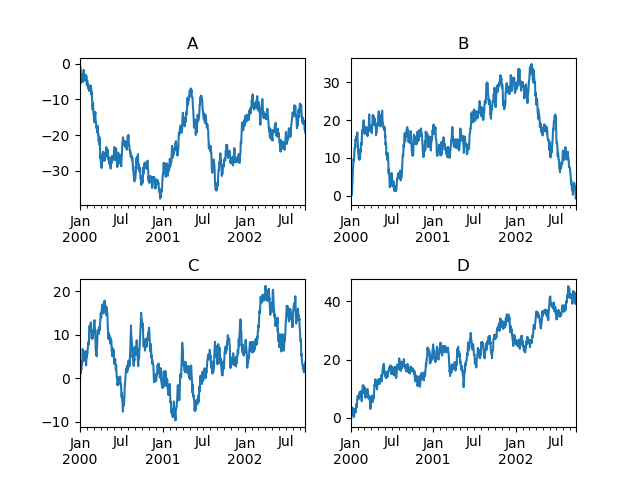### Plotting with error bars¶

Plotting with error bars is supported in `DataFrame.plot()` and `Series.plot()`.

Horizontal and vertical error bars can be supplied to the `xerr` and `yerr` keyword arguments to `plot()`. The error values can be specified using a variety of formats:

Here is an example of one way to easily plot group means with standard deviations from the raw data.

```# Generate the data
In : ix3 = pd.MultiIndex.from_arrays(
.....:     [
.....:         ["a", "a", "a", "a", "a", "b", "b", "b", "b", "b"],
.....:         ["foo", "foo", "foo", "bar", "bar", "foo", "foo", "bar", "bar", "bar"],
.....:     ],
.....:     names=["letter", "word"],
.....: )
.....:

In : df3 = pd.DataFrame(
.....:     {
.....:         "data1": [9, 3, 2, 4, 3, 2, 4, 6, 3, 2],
.....:         "data2": [9, 6, 5, 7, 5, 4, 5, 6, 5, 1],
.....:     },
.....:     index=ix3,
.....: )
.....:

# Group by index labels and take the means and standard deviations
# for each group
In : gp3 = df3.groupby(level=("letter", "word"))

In : means = gp3.mean()

In : errors = gp3.std()

In : means
Out:
data1     data2
letter word
a      bar   3.500000  6.000000
foo   4.666667  6.666667
b      bar   3.666667  4.000000
foo   3.000000  4.500000

In : errors
Out:
data1     data2
letter word
a      bar   0.707107  1.414214
foo   3.785939  2.081666
b      bar   2.081666  2.645751
foo   1.414214  0.707107

# Plot
In : fig, ax = plt.subplots()

In : means.plot.bar(yerr=errors, ax=ax, capsize=4, rot=0);
```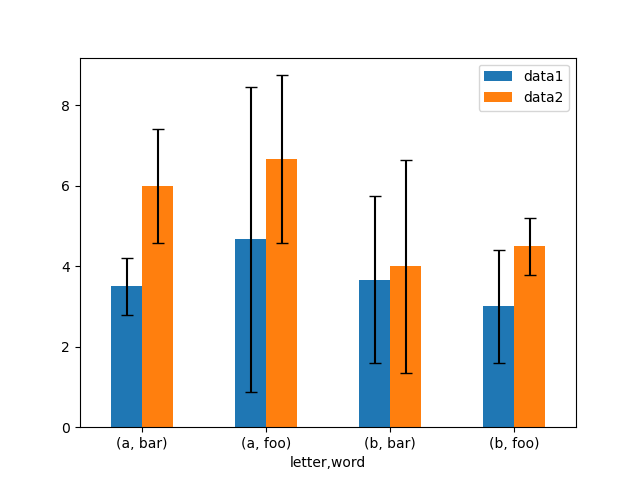Asymmetrical error bars are also supported, however raw error values must be provided in this case. For a `N` length `Series`, a `2xN` array should be provided indicating lower and upper (or left and right) errors. For a `MxN` `DataFrame`, asymmetrical errors should be in a `Mx2xN` array.

Here is an example of one way to plot the min/max range using asymmetrical error bars.

```In : mins = gp3.min()

In : maxs = gp3.max()

# errors should be positive, and defined in the order of lower, upper
In : errors = [[means[c] - mins[c], maxs[c] - means[c]] for c in df3.columns]

# Plot
In : fig, ax = plt.subplots()

In : means.plot.bar(yerr=errors, ax=ax, capsize=4, rot=0);
```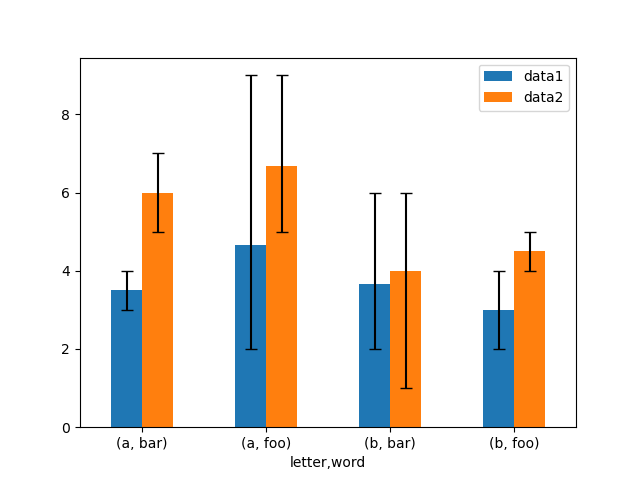### Plotting tables¶

Plotting with matplotlib table is now supported in `DataFrame.plot()` and `Series.plot()` with a `table` keyword. The `table` keyword can accept `bool`, `DataFrame` or `Series`. The simple way to draw a table is to specify `table=True`. Data will be transposed to meet matplotlib’s default layout.

```In : fig, ax = plt.subplots(1, 1, figsize=(7, 6.5))

In : df = pd.DataFrame(np.random.rand(5, 3), columns=["a", "b", "c"])

In : ax.xaxis.tick_top()  # Display x-axis ticks on top.

In : df.plot(table=True, ax=ax);
```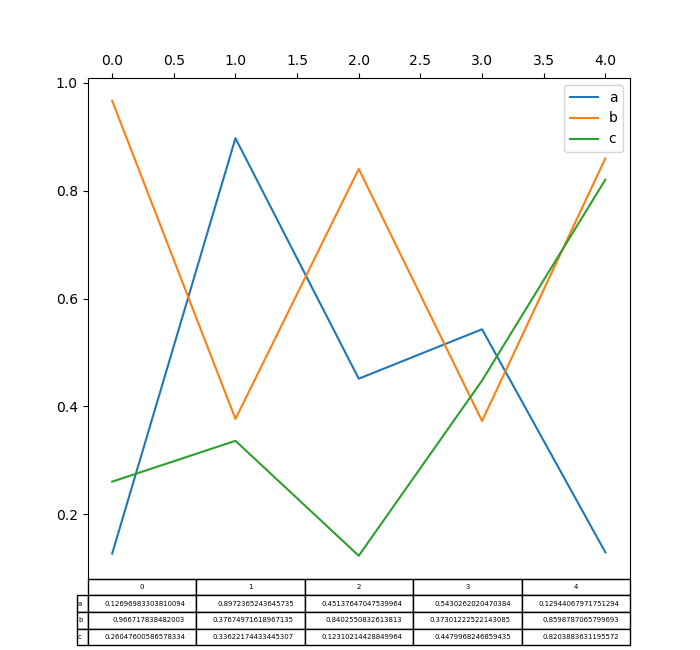Also, you can pass a different `DataFrame` or `Series` to the `table` keyword. The data will be drawn as displayed in print method (not transposed automatically). If required, it should be transposed manually as seen in the example below.

```In : fig, ax = plt.subplots(1, 1, figsize=(7, 6.75))

In : ax.xaxis.tick_top()  # Display x-axis ticks on top.

In : df.plot(table=np.round(df.T, 2), ax=ax);
```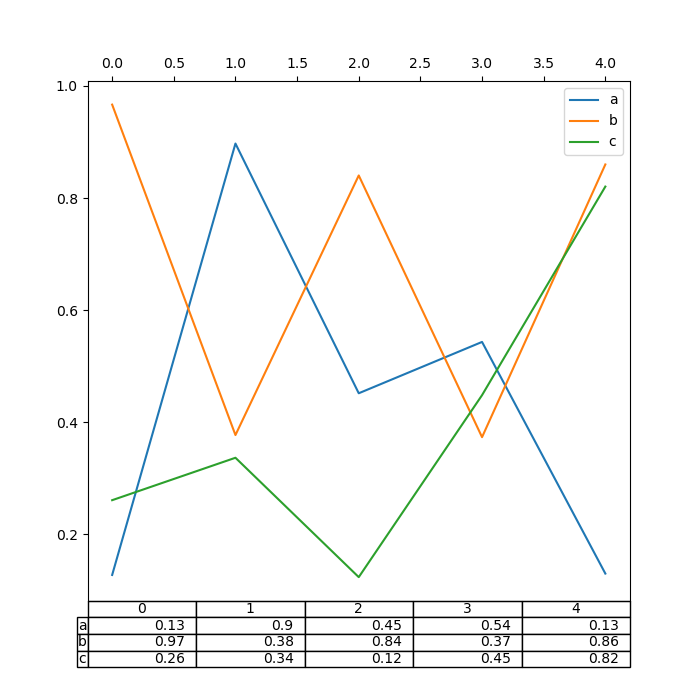There also exists a helper function `pandas.plotting.table`, which creates a table from `DataFrame` or `Series`, and adds it to an `matplotlib.Axes` instance. This function can accept keywords which the matplotlib table has.

```In : from pandas.plotting import table

In : fig, ax = plt.subplots(1, 1)

In : table(ax, np.round(df.describe(), 2), loc="upper right", colWidths=[0.2, 0.2, 0.2]);

In : df.plot(ax=ax, ylim=(0, 2), legend=None);
```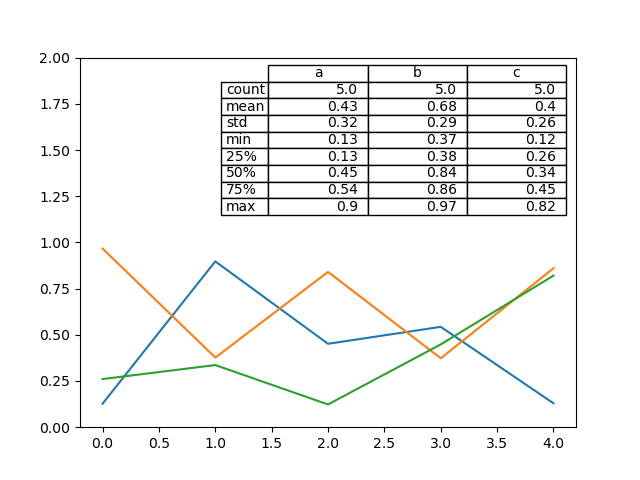Note: You can get table instances on the axes using `axes.tables` property for further decorations. See the matplotlib table documentation for more.

### Colormaps¶

A potential issue when plotting a large number of columns is that it can be difficult to distinguish some series due to repetition in the default colors. To remedy this, `DataFrame` plotting supports the use of the `colormap` argument, which accepts either a Matplotlib colormap or a string that is a name of a colormap registered with Matplotlib. A visualization of the default matplotlib colormaps is available here.

As matplotlib does not directly support colormaps for line-based plots, the colors are selected based on an even spacing determined by the number of columns in the `DataFrame`. There is no consideration made for background color, so some colormaps will produce lines that are not easily visible.

To use the cubehelix colormap, we can pass `colormap='cubehelix'`.

```In : df = pd.DataFrame(np.random.randn(1000, 10), index=ts.index)

In : df = df.cumsum()

In : plt.figure();

In : df.plot(colormap="cubehelix");
```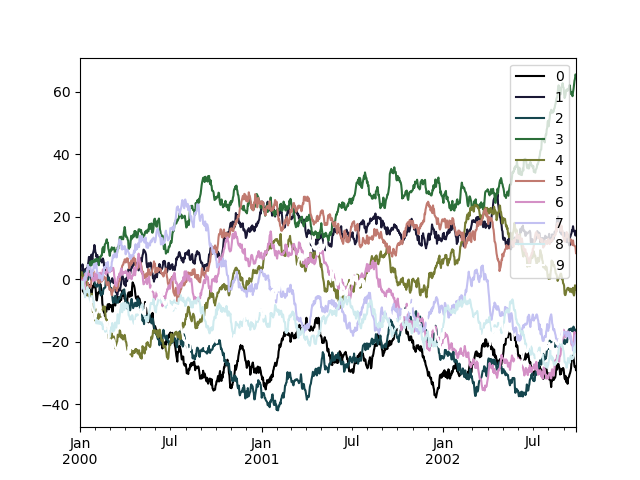Alternatively, we can pass the colormap itself:

```In : from matplotlib import cm

In : plt.figure();

In : df.plot(colormap=cm.cubehelix);
```Colormaps can also be used other plot types, like bar charts:

```In : dd = pd.DataFrame(np.random.randn(10, 10)).applymap(abs)

In : dd = dd.cumsum()

In : plt.figure();

In : dd.plot.bar(colormap="Greens");
```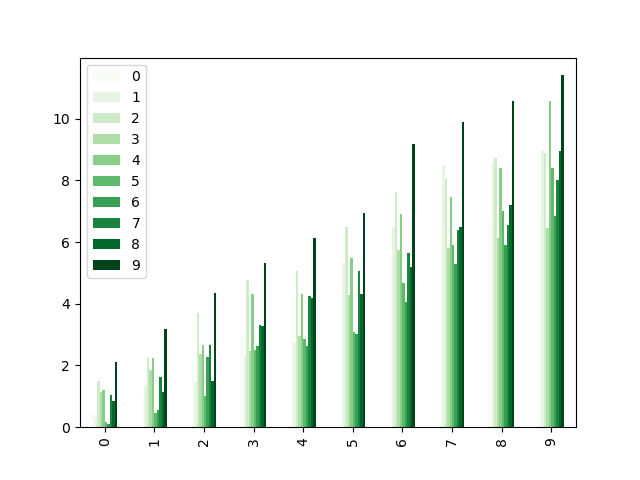Parallel coordinates charts:

```In : plt.figure();

In : parallel_coordinates(data, "Name", colormap="gist_rainbow");
```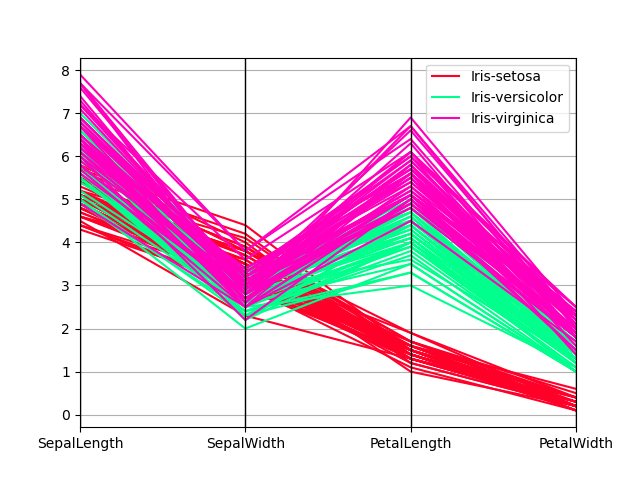Andrews curves charts:

```In : plt.figure();

In : andrews_curves(data, "Name", colormap="winter");
```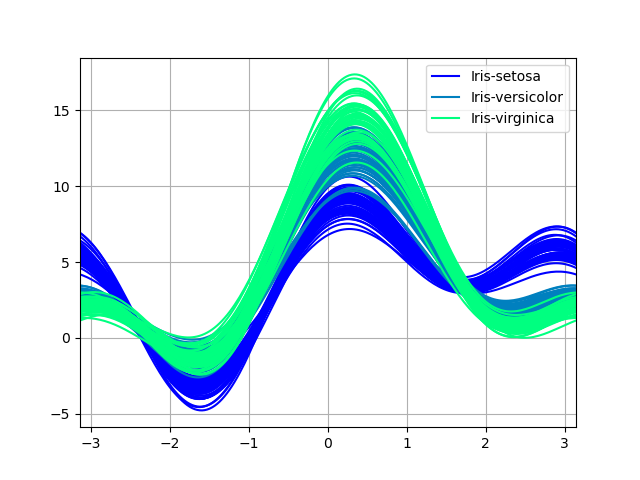## Plotting directly with matplotlib¶

In some situations it may still be preferable or necessary to prepare plots directly with matplotlib, for instance when a certain type of plot or customization is not (yet) supported by pandas. `Series` and `DataFrame` objects behave like arrays and can therefore be passed directly to matplotlib functions without explicit casts.

pandas also automatically registers formatters and locators that recognize date indices, thereby extending date and time support to practically all plot types available in matplotlib. Although this formatting does not provide the same level of refinement you would get when plotting via pandas, it can be faster when plotting a large number of points.

```In : price = pd.Series(
.....:     np.random.randn(150).cumsum(),
.....:     index=pd.date_range("2000-1-1", periods=150, freq="B"),
.....: )
.....:

In : ma = price.rolling(20).mean()

In : mstd = price.rolling(20).std()

In : plt.figure();

In : plt.plot(price.index, price, "k");

In : plt.plot(ma.index, ma, "b");

In : plt.fill_between(mstd.index, ma - 2 * mstd, ma + 2 * mstd, color="b", alpha=0.2);
```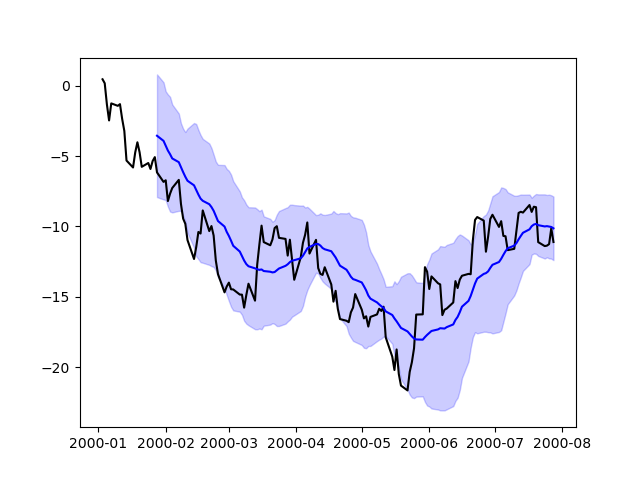## Plotting backends¶

Starting in version 0.25, pandas can be extended with third-party plotting backends. The main idea is letting users select a plotting backend different than the provided one based on Matplotlib.

This can be done by passsing ‘backend.module’ as the argument `backend` in `plot` function. For example:

```>>> Series([1, 2, 3]).plot(backend="backend.module")
```

Alternatively, you can also set this option globally, do you don’t need to specify the keyword in each `plot` call. For example:

```>>> pd.set_option("plotting.backend", "backend.module")
>>> pd.Series([1, 2, 3]).plot()
```

Or:

```>>> pd.options.plotting.backend = "backend.module"
>>> pd.Series([1, 2, 3]).plot()
```

This would be more or less equivalent to:

```>>> import backend.module
>>> backend.module.plot(pd.Series([1, 2, 3]))
```

The backend module can then use other visualization tools (Bokeh, Altair, hvplot,…) to generate the plots. Some libraries implementing a backend for pandas are listed on the ecosystem Visualization page.

Developers guide can be found at https://pandas.pydata.org/docs/dev/development/extending.html#plotting-backends